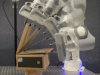### Abstract

Daily manipulation tasks are characterized by regular features associated with the task structure, which can be described by multiple geometric primitives related to actions and object shapes. Only using Cartesian coordinate systems cannot fully represent such geometric descriptors. In this article, we consider other candidate coordinate systems and propose a learning approach to extract the optimal representation of an observed movement/behavior from these coordinates. This is achieved by using an extension of Gaussian distributions on Riemannian manifolds, which is used to analyse a small set of user demonstrations statistically represented in different coordinate systems. We formulate the skill generalization as a general optimal control problem based on the (iterative) linear quadratic regulator ((i)LQR), where the Gaussian distribution in the proper coordinate systems is used to define the cost function. We apply our approach to object grasping and box-opening tasks in simulation and on a 7-axis Franka Emika robot using open-loop and feedback control. The results show that the robot can exploit several geometries to execute the manipulation task and generalize it to new situations. The results show high variation along the do-not-matter direction, while maintaining the invariant characteristics of the task in the coordinate system(s) of interest. We then tested the approach in a human-robot shared control task. Results show that the robot can modify its grasping strategy based on the geometry of the object that the user decides to grasp.

### Bibtex reference

@article{Ti23RAS,
author="Ti, B. and Razmjoo, A. and Gao, Y. and Zhao, J. and Calinon, S.",
title="A Geometric Optimal Control Approach for Imitation and Generalization of Manipulation Skills",
journal="Robotics and Autonomous Systems",
publisher="Elsevier",
year="2023",
volume="164",
pages="104413",
doi="10.1016/j.robot.2023.104413"
}